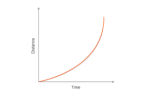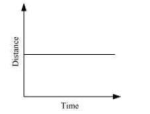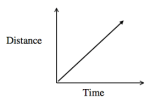# Motion & Forces BINGO - Call List

(Print) Use this randomly generated list as your call list when playing the game. There is no need to say the BINGO column name. Place some kind of mark (like an X, a checkmark, a dot, tally mark, etc) on each cell as you announce it, to keep track. You can also cut out each item, place them in a bag and pull words from the bag.

1
2
3
4
5
6
7
8
9
10
11
12
13
14
15
16
17
18
19
20
21
22
23
24
25
26
27
28
29
30
31
32
33
34
35
36
37
38
39
40
41
42
43
44
45
46
47
48
49
50
51
52
53
54
55
56
57
58
59
60
1. kg
2. Fluid friction
3. The apparent outward force acting on a rotating abject
4. a plane inclined at an angle to the horizontal.
5. The quantity of matter in an object
6.7. Acceleration
8. This acts on a mass to create the force of weight
9. combination of the standard three additive primary colors
10. Speed
11. A negative change in velocity
12. The rate at which velocity is changing
13. Sliding Friction
14. Net force
15. Negative acceleration
16. Newton
17.18. the property of matter that measures its resistance to acceleration
19. Force that is always present & keeps things upright
20. The unit of measurement for forces
21. when the net force on an object is zero
22. The sum of all forces acting on an object
23. p=m/V
24. A force that counteracts motion
25. m/s
26. states that the force (F) needed to extend or compress a spring by some distance X is proportional to that distance.
27. Velocity
28. Unbalanced force
29. Balanced Force
30. velocity at a specific time 't'
31. the frictional force that prevents two surfaces from sliding past each other
32. also known as the law of inertia
33. states, “The force acting on an object is equal to the mass of that object times its acceleration.”
34. A push or a pull
35. speed + direction
36. the resistance force that slows down the motion of a rolling ball or wheel.
37. Mass
38. states that for every action, there is an equal and opposite reaction.
39. each of the digits of a number that are used to express it to the required degree of accuracy, starting from the first nonzero digit.
40. Static friction
41. Universal Gravitational Law
42. Distance
43. The inward pulling force on a rotating object
44. Rise over run
45. An objects resistance to change its motion
46. Gravity
47. Rolling friction
48. An object at rest remains at rest; and if in motion will remain in motion in a straight line; unless a force acts on it
49. The unit of measurement for force
50. The pull of gravity on an object's mass (mg=)
51.52. The change in angular displacement divided by time
53. Magnitude of distance traveled divided by time taken
54. An objects resistance to change in its motion
55. Force
56. Friction
57. A positive change in velocity
58. the gravitationally curved path of an object around a point in space
59. Positive acceleration
60. a change in position of an object